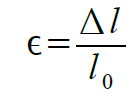# Strain

## What is strain?

The strain is a measure of the relative change in length.
"Relative change in length" means the increase or decrease of the length Δl relative to the original length l0:
The formula for the strain is ε.The elongation ε = Δl / 10 is positive in the case of an increase in the original strain (and is referred to as "stretching"), or is negative (and is referred to as "compression")

## Where does strain come from?

Strain is caused, for example, by the action of force on a material. However, it can also be caused by temperature, or by various physical effects.
When stretching in response to a force, one speaks of "deformation".
If the strain is caused by a change in the temperature, this is called thermal expansion.
By the magnetostrictive effect and by the piezoelectric effect, strains are caused by a magnetic or electric field.

## How to calculate strain?

We know materials which are particularly deformed by the action of a force, e.g. rubber, or particularly little deformation, e.g. steel.
In addition, there are materials which are particularly expansive under the influence of temperature, e.g. aluminum, or very little, such as titanium.
The relationship between strain and its cause is described by material coefficients (often also as a "module", or even as a "constant"):

 material coefficient Description modulus of elasticity Describes the relationship between the strain and the mechanical load Thermal expansion coefficient Describes the relationship between strain and temperature Piezoelectric module Describes the relationship between the strain and the electric field strength Magnetostrictive module Describes the relationship between the strain and the magnetic field strength

## Which unit has strain?

The unit of strain is meters per meter: [m/m]
Since the change in length Δl is very small compared to the original length l0, the strain ε is often given in microns per meter: [μm / m].
If the unit m/m is reduced, 10-6 or "parts per million" (ppm) remain. The elongation is also expressed in percent (‰) or in percent (%).
In the US, the unit "microepsilon" or "microstrain" [με] stands for 1 μm/m
10-6m/m=1ppm=1μm/m=1μϵ=10-3‰=10-4%

## How big is strain?

A steel rod with a length of one meter expands by 12 micrometers per degree Celsius temperature change.
An aluminum rod expands by 23 micrometers per meter per degree Celsius, almost twice as much compared to steel.
A force sensor is loaded up to strain of 1000 micrometers per meter (1 per thousand).
If an aluminum sensor is used, then 44 degrees Celsius will cause as much strain as a 100% load of a force sensor (1000um/m).
A force sensor made of aluminum undergoes an elongation of 10 μm / m at a load of 1%, or at a temperature change of 0.44 ° C.

## How to distinguish between thermal expansion and mechanical strain?

The magnitudes for thermal expansion and strain due to a force make it clear that the thermal expansion is a large source of error for a force sensor.
First, one can not distinguish heat expansion from any other type of strain. However, most materials extend uniformly in all directions of the space, while mechanical deformation occurs mainly in the direction of the mechanical load. In the other directions there is usually even a deformation with an opposite sign instead of the effect of "transverse contraction".
These (and other) effects are used to compensate for temperature-related errors.

## What is the temperature-induced drift for measurements with strain gauges?

The use of the "full bridge circuit", the "self-compensation" and "drift matching" with resistors provide the lowest possible drift.
After the compensation of temperature - related faults, the "apparent" strain due to temperature change is reduced to an order of magnitude of
0.01μm/m/°C ... 1.0μm/m/°C.

## How to measure strain?

The strain can be measured directly

• With strain gauges ("electrical"),
• With strain gauges ("optical"),
• With stress-optical methods,
• With zipper.

## What is the smallest strain?

The following table provides an overview of the smallest achievable resolutions:

 Resolution Technology ca. 0,01μm/m ... 0,1μm/m Metal foil strain gauges ca. 0,001 μm/m ... 0,01 μm/m Semiconductor strain gauges ca. 100 μm/m photoelasticity ca. 100 μm/m varnish

## What is the difference between length measurement and strain measurement?

In principle, one can calculate the strain from a length measurement and, conversely, a length change can be calculated from a strain measurement.
The strain measurement is mainly characterized in that an enlargement or reduction of the strain gauge has no direct influence on the sensitivity of the measurement:
The strain gauge always measures the average (average) strain below its surface.
A smaller strain gauge is particularly suited to refine the local resolution (e.g., near notches), while a large strain gauge primarily provides a mean value over locally varying strain (e.g., fiber composites).
"There is no longer any measuring signal" due to longer measuring grids.

## What are the possibilities to "enlarge" the measuring signal?

To a first approximation, all metal foil strain gauges have the same "sensitivity" (the same "k-factor").
The signal level can only be influenced by the "bridge circuit" as a "full" or "half bridge" and by the bridge supply voltage.
Due to the self-heating or the power loss of the strain gauge, the feeding voltage can not be increased as desired.